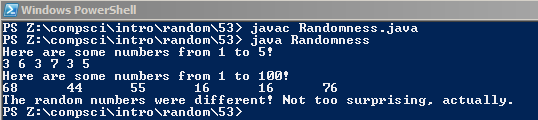# Assignemnt #53 and Randomness

## Code

```///Name: Ben Borglin
///Period: 6
///Program name: Randomness
///file name: Randomness.java
///Date Finsihed: 10/16/15

import java.util.Random;

public class Randomness
{
public static void main( String[] args )
{
Random r = new Random();

int x = 1 + r.nextInt(10);

System.out.println( "Here are some numbers from 1 to 5!" );
System.out.print(3 + r.nextInt(5) + " " );
System.out.print(3 + r.nextInt(5) + " " );
System.out.print(3 + r.nextInt(5) + " " );
System.out.print(3 + r.nextInt(5) + " " );
System.out.print(3 + r.nextInt(5) + " " );
System.out.print(3 + r.nextInt(5) + " " );
System.out.println();

///With +3 infront it shows a range of 3-7 (3 + 5 and 3 - 5)
///Changing the seed to 4545454 it makes the random numbers be the same everytime

System.out.println( "Here are some numbers from 1 to 100!" );
System.out.print( 1 + r.nextInt(100) + "\t" );
System.out.print( 1 + r.nextInt(100) + "\t" );
System.out.print( 1 + r.nextInt(100) + "\t" );
System.out.print( 1 + r.nextInt(100) + "\t" );
System.out.print( 1 + r.nextInt(100) + "\t" );
System.out.print( 1 + r.nextInt(100) + "\t" );
System.out.println();

int num1 = 1 + r.nextInt(10);
int num2 = 1 + r.nextInt(10);

if ( num1 == num2 )
{
System.out.println( "The random numbers were the same! Weird." );
}
if ( num1 != num2 )
{
System.out.println( "The random numbers were different! Not too surprising, actually." );
}
}
}

```

### Picture of the output